Next: References Up: GEOMETRY-BASED DECON Previous: A model for convolution

## Heavy artillery

In Figure 6, we can see that events remain which look suspiciously like multiple reflections. Careful inspection of the data (rapid blinking on a video screen) convinced me that the problem lay in imperfect modeling of depth-variable velocity. It is not enough to use a depth-variable velocity in the NMO (a constant velocity was used in Figure 6), because primary and multiple reflections have different velocities at the same time. I used instead a physical technique called diffraction" (explained in detail in IEI) to make the regressors. Instead of simply shifting on the time axis, diffraction shifts on the depth axis, which results in subtle changes in hyperbola curvature.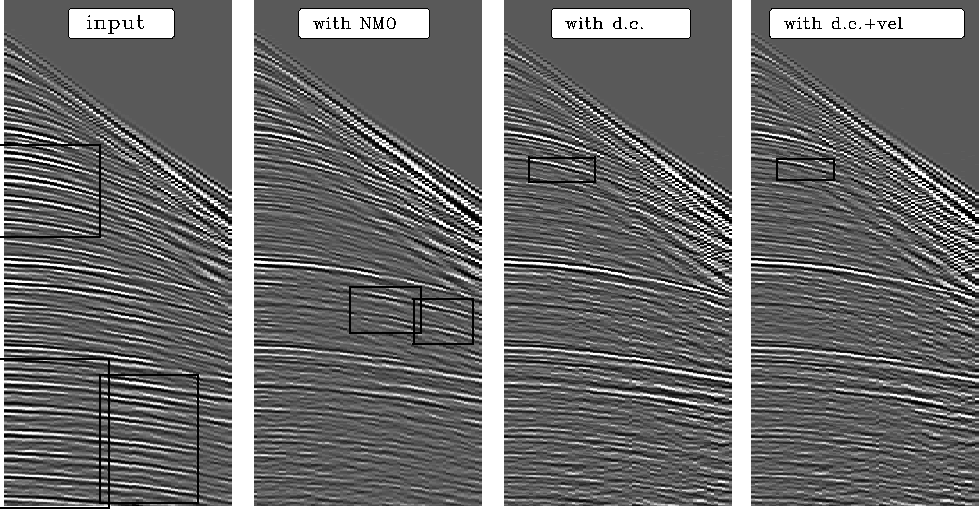veld
Figure 9
Left is the original data. Next is the result of using NMO in the regressors. Next, the result of downward continuation in the regressors. On the right, velocity scans were also used. Rectangles outline certain or likely multiple reflections.

The downward-continuation result is significantly better than the NMO result, but it does contain some suspicious reflections (boxed). My final effort, shown on the right, includes the idea that the data contains random noise which could be windowed away in velocity space. To understand how this was done, recall that the basic model is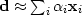, where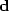is the left panel,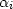are constants determined by least squares, andare the regressors, which are panels likebut delayed and diffracted. Let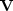denote an operator that transforms to velocity space. Instead of solving the regression,I solved the regression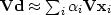and used the resulting values ofin the original (t,x)-space. (Mathematically, I did the same thing when making Figure.) This procedure offers the possible advantage that a weighting function can be used in the velocity space. Applying all these ideas, we see that a reflector remains which looks more like a multiple than a primary.

 A regression () can be done in any space. You must be able to transfer into that space (that is, to make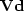and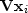) but you do not need to be able to transform back from that space (you do not need). You should find thein whatever space you are able to define the most meaningful weighting function.

A proper industrial strength'' attack on multiple reflections involves all the methods discussed above, wave-propagation phenomena described in IEI, and judicious averaging in the space of source and receiver distributions.Next: References Up: GEOMETRY-BASED DECON Previous: A model for convolution
Stanford Exploration Project
10/21/1998